LCL for X chart =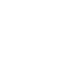UCL for X chart =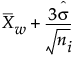LCL for S-chart =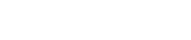UCL for S-chart =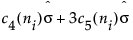Center line for S-chart: By default, the center line for the ith subgroup (where 3 is the sigma multiplier) indicates an estimate of the expected value of si. This value is computed as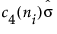, where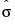is an estimate of σ.
σ = process standard deviation
ni = sample size of ith subgroup
c4(n) is the expected value of the standard deviation of n independent normally distributed variables with unit standard deviation
c5(n) is the standard deviation of the standard deviation of n independent observations from a normal population with unit standard deviation
N is the number of subgroups for which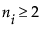si is the sample standard deviation of the ith subgroup

Help created on 9/19/2017